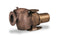The pump is the main part of any pool, especially important for commercial pools. A properly sized pump means adequate flow which is key to proper filtration and helps to keep the pool safe for bathers. When a commercial pool pump is sized incorrectly, it can mean costly downtime and potentially affect the healthy and safety of many people.

1. To determine the correct size commercial pool pump, there are a few calculations required. First you need to determine the total dynamic head (TDH) which is measured in feet. TDH represents the amount of resistance the pool pump has to overcome in order to circulate water through the pool's plumbing. Resistance comes from the pipe size, any plumbing elbows, reducers and anything that creates pressure within the plumbing.

2. To calculate the total dynamic head, take a reading of the filter's pressure (PSI) while the filter is clean and running. Multiply the PSI reading by 2.31 and this will give you the TDH. The formula is PSI x 2.31 = TDH.

3. If you don't already know the number of gallons in the pool, this will be the next calculation necessary to size the pump. There are several formulas for calculating this based on the shape, dimensions and average depth. For rectangular pools, you would multiply length x width x average depth (in feet) x 7.5. For round pools, multiply diameter x diameter x average depth (in feet) x 5.9. For oval pools, multiply long diameter x short diameter x average depth (in feet) x 5.9.

4. This calculation is trickier for free-form pools but you can first calculate the pool's square footage by drawing a diagram of the pool (to scale) on graph paper. Count all of the squares, also accounting for the partially filled ones (in other words, estimate how many full squares the partial ones would equal). This will give you a pretty accurate estimate of the square feet; multiply square feet x average depth x 7.48 to get the number of gallons.

5. Once you have calculated the gallons of water in the pool, the next step is determining the desired flow rate. You would divide the number of gallons by turnover time (hours) to get the gallons per hour (gph). Take that number and divide by 60 to get the gallons per minute (gpm). For example, if you have a 100,000 gallon pool and want to turn over all of the water in 8 hours, your formula would be 100,000/8 = 12500 gph and then 12500/60 = about 208 gpm. In this example, your pool pump would ideally be sized properly to output 208 gpm.

6. Next you will need to take a look at the pump performance chart provided by the manufacturer. This chart is a graph showing the gallons per minute based on the total dynamic head in feet. Follow the TDH line to the intersection with the desired gpm rating and then refer to the manufacturer's key to see which model commercial pump meets the TDH and gpm you have calculated

If you have any other questions about pool and spa products please do let us know - we are here to help!